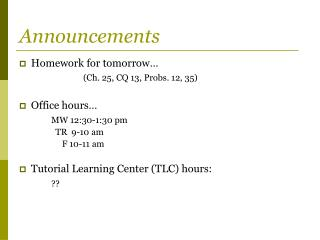DownloadDownload PresentationAnnouncements

# Announcements

Télécharger la présentation## Announcements

- - - - - - - - - - - - - - - - - - - - - - - - - - - E N D - - - - - - - - - - - - - - - - - - - - - - - - - - -
##### Presentation Transcript

1. Announcements • Homework for tomorrow… (Ch. 25, CQ 13, Probs. 12, 35) • Office hours… MW 12:30-1:30 pm TR 9-10 am F 10-11 am • Tutorial Learning Center (TLC) hours: ??

2. Chapter 25 Electric Forces & Charges (Coulomb’s Law)

3. Newton’s Law of Gravitation, revisited.. where Notice: • Mass is always positive. • Gravity is alwaysattractive. m2 r m1

4. Coulomb’s Law.. where Notice: • Charges can be positive or negative. • Force can be attractive or repulsive. q2 r q1

5. Coulomb’s Law - restrictions charges must be small compared to their separation (“point-like”) OK Not OK

6. Quiz Question 1 Two uniformly charged spheres are firmly fastened to and electrically insulated from frictionless pucks on an air table. The charge on sphere 2 is three times the charge of sphere 1. Which force diagram correctly shows the magnitude and direction of the electrostatic forces:

7. Coulomb’s Law and Superposition • Q: What is the ? q1 q2 q3

8. Coulomb’s Law and Superposition • Q: What is the ? • A: q1 q2 q3

9. Quiz Question 2 Two protons (p1 and p2) and an electron (e) lie on a straight line as shown. The direction of the net force on p1 ,p2 , and e, respectively, are: • left, right, left • left, right, right • right, left,left • right, left, right • right, right, left p1 p2 e

10. Prob. 25.16 What is the net electric force on chargeAin Figure EX25.16?

11. Prob. 25.37 What is the force F on the 5.0 nC charge in Figure P25.37? Give your answer as a magnitude and an angle measured cw or ccw (specify which) from the + x-axis.